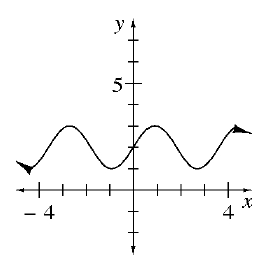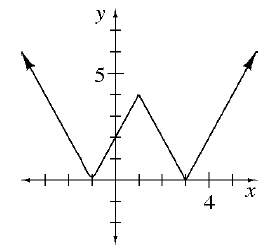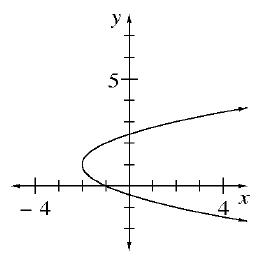### Home > CCAA8 > Chapter 2 Unit 2 > Lesson CCA: 2.1.1 > Problem2-9

2-9.

1.Do all of these graphs have one output value for every input value?

1.1.1. For each graph above, state the domain and range.

• The domain of a graph is its possible $x$-values.

All real numbers.

The range of a graph is its possible $y$-values.

$1\le y\le3$